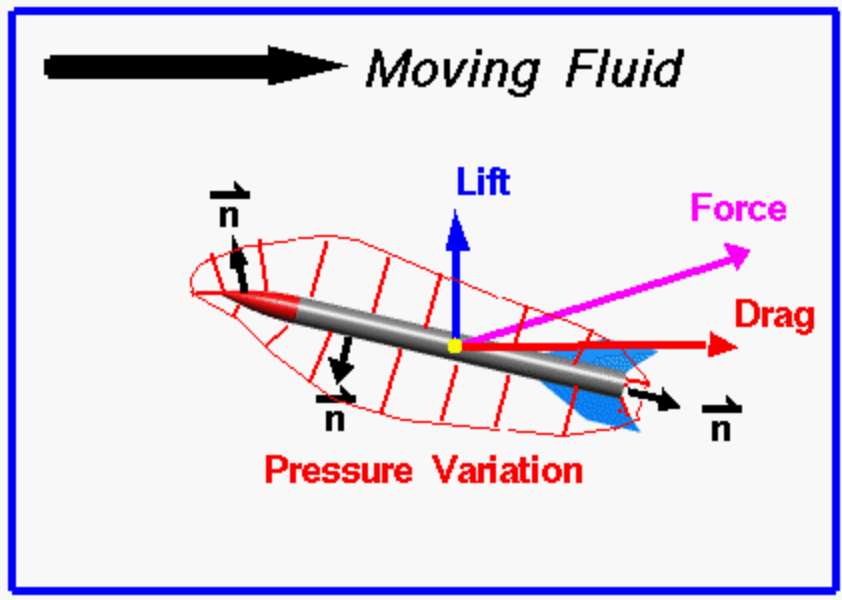# Rocket aerodynamics

## Aerodynamic forcesFor a small area of the rocket’s surface, the total aerodynamic force depends on the air pressure acting upon it:

$$p = \frac{F}{A} \Rightarrow F = p\cdot A$$

This force acts perpendicular to the surface. A positive pressure acts inwards on the rocket, and a negative one pulls away from it. By summing up all these contributions over the entire rocket we can obtain the total aerodynamic force acting on the rocket:

$$\vec F_{a} =-\sum_{i=0}^N p_iA_i\vec n_i$$

Where $\vec n_i$ are the outward-pointing surface normals.
If we make the areas infinitely small, this becomes an integral:

$$\vec F_{a} = \lim_{N\to\infty, A_i\to 0} -\sum_{i=0}^N p_iA_i \vec n_i= -\oint p_i\vec n_i \cdot \text{d}A$$

Since the drag force is defined as being the component of $\vec F_{aero}$ in the direction of fluid motion, and the lift force is perpendicular to that, we can calculate them as follows:
$$\vec F_{D} = \vec F_{a} \cdot \frac{\vec u}{||\vec u||}$$
$$\vec F_{L} = \vec F_{a}-\vec F_{D}$$

## Factors affecting drag

There are many factors that affect aerodynamic forces. In the case of a rocket we are mostly interested in drag, since lift is usually quite small.

• Geometry: the shape and size of the rocket directly affects the form drag created by the pressure variations around the rocket. The smoothness of the rocket’s surface affect the rocket’s skin drag.
• Motion of the air: lift and drag depend on the square of the relative velocity between the rocket and the air. The inclination (angle of attack) of the rocket also affects these. If the rocket is traveling at transonic or supersonic speeds, shock waves are formed which produce and additional drag component called wave drag.
• Properties of the air: lift and drag depend directly on the mass (and therefore density) of the flow around the rocket. Two other factors also come in to play: the viscosity and compressibility of the air affect skin drag and wave drag respectively.

All these factors converge into two mathematical equations called the Lift Equation and the Drag Equation. With the these equations we can predict how much aerodynamic force is generated by a given body moving at a given speed through a given fluid.

### Air density and aerodynamic forces

The aerodynamic force acting on the rocket depends on the air density around the rocket:
$$F_a \propto \rho u^2 = 2q$$
Where $q$ is the dynamic pressure, defined as $q = \frac{\rho u^2}{2}$.
Of course the atmospheric density varies with altitude:
$$\rho = \frac{p_0 M}{RT} \left( 1-\frac{Lh}{T_0}\right)^{gM/RL}$$

• $p_0 =$ sea level standard atmospheric pressure, $101325$ Pa
• $T_0 =$ sea level standard temperature, $288.15$ K
• $g =$ earth-surface gravitational acceleration, $9.08665 m/s^2$
• $L =$ temperature lapse rate, $0.0065$ K/m
• $R =$ universal gas constant, $8.31447 \frac{\text J}{\text{mol K}}$
• $M =$ molar mass of dry air, $0.0289654$ kg/mol
• $h =$ altitude in meters above sea level
• $T =$ absolute temperature, approximated by $T = T_0-Lh$ below $\sim18$ km